# The Traveling Salesperson Problem¶

Consider the Traveling Salesperson Problem (or TSP):

Given a set of cities and the distance between each pair of cities, what is the shortest possible tour that visits each city exactly once, and returns to the starting city?

In this notebook we will develop some solutions to the problem, and more generally show how to think about solving problems. The algorithms developed here are used in serious applications that millions of people rely on every day.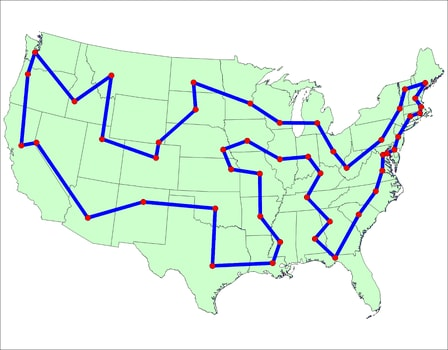An example tour

Do we understand the problem statement well enough to program a solution? Let's check:

• Given a set of cities
A Python set could represent a set of cities. An individual city might be just an integer index, or it might be (x, y) coordinates.
• ... and the distance between each pair of cities:
We could use either a function, distance(A, B), or a table, distance[A, B].
• ... what is the shortest possible tour
A tour is a sequential order in which to visit the cities; a function shortest_tour(tours) should find the one that minimizes tour_length(tour), which is the sum of the distances between adjacent cities in the tour.
• ... that visits each city once and returns to the starting city
Make sure a tour doesn't re-visit a city (except returning to the start).

I don't yet have all the answers, but I'm ready to attack the problem.

In :
# Imports used in this notebook. This is Python 3 on Jupyter with matplotlib.
%matplotlib inline
import matplotlib.pyplot as plt
import random
from time        import clock
from itertools   import permutations, combinations
from functools   import lru_cache as cache
from collections import Counter
from statistics  import mean, median


# Exhaustive Search Algorithm: exhaustive_tsp¶

Let's start with an algorithm that is guaranteed to solve the problem, although inefficiently:

Exhaustive Search Algorithm: Generate all possible tours of the cities, and choose the shortest tour (the one with minimum tour length).

My design philosophy is to first write an English description of the algorithm (as above), then write Python code that closely mirrors the English description. This will probably require some auxilliary functions and data structures; just assume they exist; put them on a TO DO list, and eventually define them with the same design philosophy:

In :
def exhaustive_tsp(cities):
"Generate all possible tours of the cities and choose the shortest tour."
return shortest_tour(alltours(cities))

def shortest_tour(tours): return min(tours, key=tour_length)

# TO DO: Data types: City, Cities, Tour; Functions: alltours, tour_length


This gives us a good start; the Python code closely matches the English description. Now for the TO DO list.

Tours: A tour that starts in city 1, moves to 2, then 3, then back to 1 will be represented by (1, 2, 3). Any valid tour of a set of cities will be a permutation of the cities. That means we can implement alltours with the built-in permutations function (from the itertools module). The length of a tour is the sum of the distances between adjacent cities in the tour—the sum of the lengths of the links between cities in the tour.

Cities: the only thing we need to know about a city is its distance to other cities. We don't need to know the city's name, population, best restaurants, or anything else. We'll assume the distance between two cities is the Euclidean distance, the straight-line distance between points in a two-dimensional plane. So I want City(300, 100) to be the city with x-coordinate 300 and y coordinate 100. At first glance it seems like Python does not have a builtin type for a point in the two-dimensional plane, but actually there is one: complex numbers. I'll implement City with complex, which means the distance between two cities, distance(A, B), is the absolute value of the vector difference between them. I'll also define Cities(n) to make a set of n random cities. I want Cities(n) to be reproducible (to return the same result when called with the same arguments), so I provide an optional argument that sets random.seed.

In :
alltours = permutations

def tour_length(tour):
"""The total of distances between each pair of consecutive cities in the tour.
This includes the last-to-first, distance(tour[-1], tour)"""
return sum(distance(tour[i - 1], tour[i])
for i in range(len(tour)))

City = complex

def distance(A, B): return abs(A - B)

def Cities(n, seed=123, width=999, height=666):
"Make a set of n cities, sampled uniformly from a (width x height) rectangle."
random.seed((n, seed))
return frozenset(City(random.randint(1, width), random.randint(1, height))
for c in range(n))


# A solution!¶

Now we're ready: exhaustive_tsp can find a tour for a set of cities:

In :
exhaustive_tsp(Cities(9))

Out:
((158+421j),
(297+397j),
(832+102j),
(872+207j),
(817+315j),
(939+600j),
(620+498j),
(163+639j),
(31+501j))

Quick, is that the shortest tour? I can't tell. But this should help:

## Visualizing results: plot_tour and do¶

In :
def plot_tour(tour, style='bo-'):
"Plot every city and link in the tour, and highlight start city."
if len(tour) > 1000: plt.figure(figsize=(15, 10))
start = tour[0:1]
plot_segment(tour + start, style)
plot_segment(start, 'rD') # start city is red Diamond.

def plot_segment(segment, style='bo-'):
"Plot every city and link in the segment."
plt.plot([X(c) for c in segment], [Y(c) for c in segment], style, clip_on=False)
plt.axis('scaled')
plt.axis('off')

def X(city): "X coordinate."; return city.real
def Y(city): "Y coordinate."; return city.imag

In :
plot_tour(exhaustive_tsp(Cities(9)))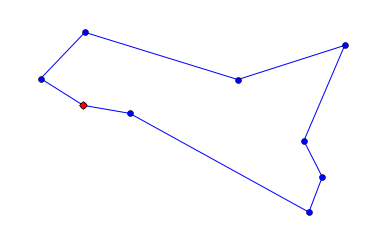That looks much better! It certainly looks like the shortest possible tour, although I can't prove it.

Vocabulary note: A segment is a portion of a tour that does not loop back to the start. The segment (1, 2, 3) has only two links, 1-2 and 2-3, whereas the tour (1, 2, 3) has three links, because it includes the link back to the start, 3-1.

One more convenience: the function do runs a TSP algorithm on a set of cities, plots the tour, asserts it is valid, and prints summary information.

In :
def do(algorithm, cities):
"Apply a TSP algorithm to cities, plot the result, and print info."
t0   = clock()
tour = algorithm(cities)
t1   = clock()
assert Counter(tour) == Counter(cities) # Every city appears exactly once in tour
plot_tour(tour)
print("{}: {} cities ⇒ tour length {:.0f} (in {:.3f} sec)".format(
name(algorithm), len(tour), tour_length(tour), t1 - t0))

def name(algorithm): return algorithm.__name__.replace('_tsp', '')

In :
do(exhaustive_tsp, Cities(9))

exhaustive: 9 cities ⇒ tour length 2450 (in 1.049 sec)## Optimization: non-redundant alltours¶

We said there are n! tours of n cities, and thus 6 tours of 3 cities:

In :
list(alltours({1, 2, 3}))

Out:
[(1, 2, 3), (1, 3, 2), (2, 1, 3), (2, 3, 1), (3, 1, 2), (3, 2, 1)]

But this is redundant: (1, 2, 3), (2, 3, 1), and (3, 1, 2) are three ways of describing the same tour. So let's arbitrarily say that a tour must start with the first city in the set of cities. While we're redefining alltours, we'll take the opportunity to define a tour as a list rather than a tuple. It doesn't matter now, but I anticipate wanting to represent partial tours, to which we will append cities one by one; appending can be done to lists, but not tuples.

In :
def alltours(cities):
"Return a list of non-redundant tours (permutations of cities)."
start, *others = cities
return [[start] + Tour(perm) for perm in permutations(others)]

Tour = list  # A Tour is a list of cities


We can verify that for 3 cities there are now only 2 tours, and that exhaustive_tsp can now do 10 cities in about the time it took to do 9 before:

In :
alltours({1, 2, 3})

Out:
[[1, 2, 3], [1, 3, 2]]
In :
do(exhaustive_tsp, Cities(10))

exhaustive: 10 cities ⇒ tour length 2720 (in 1.466 sec)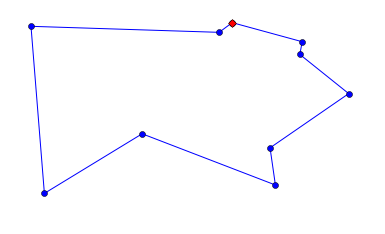# General Strategies¶

It takes Exhaustive Search 1 or 2 seconds to solve a 10-city problem. Since it looks at all permutations, an 11-city problem would take about 11 times longer, and a 15-city problem would take days. There must be a better way ...

To get inspired, here are some general strategies for algorithm design:

• Brute Force Strategy: The strategy used for exhaustive_tsp; as Ken Thompson says, "when in doubt, use brute force."
• Approximation Strategy: If it is too hard to find a precise, optimal solution, consider finding an approximate, suboptimal solution.
• Greeedy Strategy: To complete a multiple step problem, first do the step that has the best gain. Repeat.
• Iterative Improvement Strategy: Use an existing algorithm to create a solution, then have another algorithm improve the solution.
• Ensemble Strategy: Apply a set of algorithms to the problem, and pick the best solution.
• Divide and Conquer Strategy: Split the problem in half, solve each half, and combine the two partial solutions.
• Stand on the Shoulders of Giants Strategy: Find out what other people have done, and copy (or modify).

Let's apply these strategies to develop some TSP algorithms.

# Nearest Neighbor Algorithm: nn_tsp¶

Nearest Neighbor Algorithm: Start at some city; at each step extend the tour by moving from the previous city to its nearest neighbor that has not yet been visited.

This is an instance of both the approximation strategy and the greedy strategy, where we are being greedy about choosing the shortest link to a neighbor. So now, instead of considering all n! tours, we incrementally build a single tour. In more detail:

• Start at some city (pass the start city as an argument, or if None, use the first city in the set)
• ... at each step extend the tour (using tour.append)
• ... by moving from the previous city (C)
• ...to its nearest neighbor (as given by the function nearest_neighbor)
• ...that has not yet been visited (I will maintain a set of unvisited cities)
In :
def nn_tsp(cities, start=None):
"""Start the tour at the given start city (default: first city);
at each step extend the tour by moving from the previous city
to its nearest neighbor that has not yet been visited."""
C = start or first(cities)
tour = [C]
unvisited = set(cities - {C})
while unvisited:
C = nearest_neighbor(C, unvisited)
tour.append(C)
unvisited.remove(C)

def first(collection): return next(iter(collection))

def nearest_neighbor(A, cities):
"Find the city in cities that is nearest to city A."
return min(cities, key=lambda C: distance(C, A))


While exhaustive_tsp was limited to about a dozen cities, this algorithm can do thousands of cities in less than a second:

In :
do(nn_tsp, Cities(2000))

nn: 1998 cities ⇒ tour length 33688 (in 0.510 sec)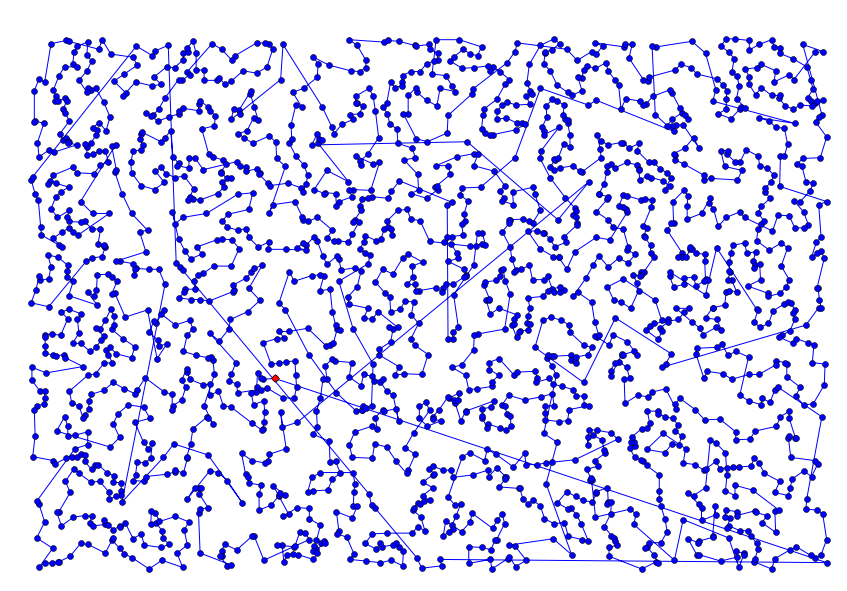(Note: I asked for 2000 cities but only got 1998 distinct ones—sometimes the random number generator produces the exact same city.)

In :
do(nn_tsp, Cities(10))

nn: 10 cities ⇒ tour length 2792 (in 0.000 sec)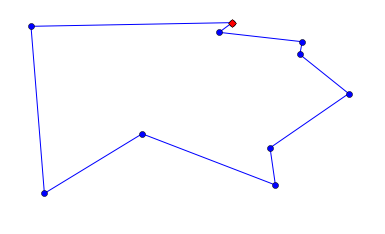On Cities(10), nn_tsp took almost no time, but the tour is not optimal: it is 3% longer than optimal. But that will vary, depending on the set of cities, and also depending on the starting city. So that gives me an idea: Just as with buying lottery tickets, we can improve our chance of winning by trying more often.

## Repetitive Nearest Neighbor Algorithm: rep_nn_tsp¶

Repetitive Nearest Neighbor Algorithm: Run the nearest neighbor algorithm repeatedly, each time with a different start city, and pick the resulting tour with the shortest total distance.

This is an instance of the ensemble strategy, because providing a different paramater to the function each time is like using a different algorithm each time. Which starting cities should we pick? I'll define a function to randomly sample the cities (and for reproducibility I'll give it a seed argument, as I did with Cities). The parameter k says how many cities to sample.

In :
def rep_nn_tsp(cities, k=25):
"Repeat nn_tsp starting from k different cities; pick the shortest tour."
return shortest_tour(nn_tsp(cities, start) for start in sample(cities, k))

def sample(population, k, seed=42):
"Return a list of k elements sampled from population. Set random.seed."
if k >= len(population):
return population
else:
random.seed((k, seed))
return random.sample(population, k)


Let's try it:

In :
do(rep_nn_tsp, Cities(10))

rep_nn: 10 cities ⇒ tour length 2720 (in 0.000 sec)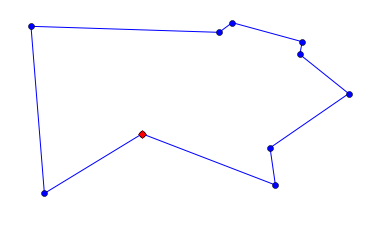In :
do(rep_nn_tsp, Cities(2000))

rep_nn: 1998 cities ⇒ tour length 32382 (in 12.480 sec)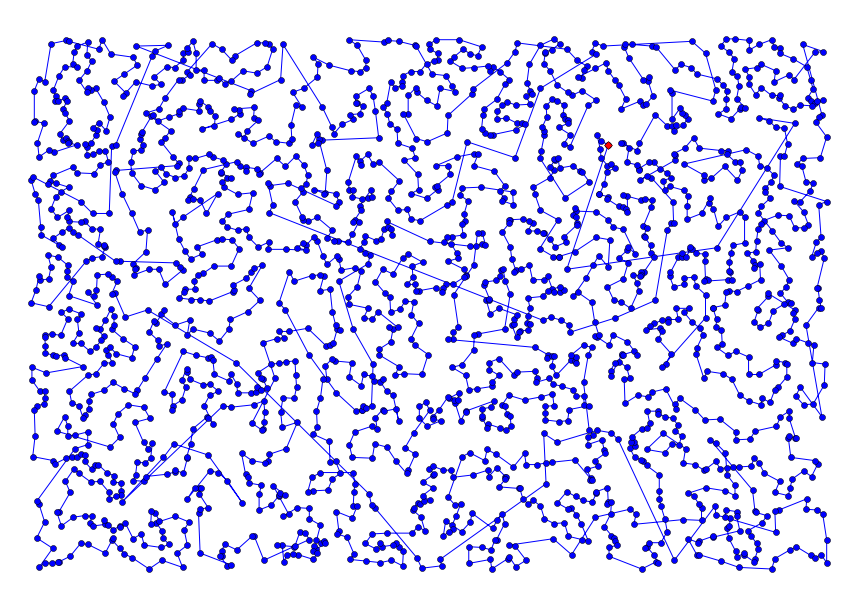That's encouraging; it found the optimal tour for Cities(10) and it improved the Cities(2000) tour by 4%, although it does take k times longer to run.

# Real Cities¶

Random cities are boring to look at; I thought it would be fun to work on some real cities. I found a web page (now 404, but a copy is here) that lists coordinates of over 10,000 cities in the USA, in this format:

[TCL]  33.23   87.62  Tuscaloosa,AL
[FLG]  35.13  111.67  Flagstaff,AZ
[PHX]  33.43  112.02  Phoenix,AZ



I define the function parse_cities to take an iterable of lines and build a City out of each line that matches the format (excluding Alaska and Hawaii).

In :
def continental_USA(line):
"Is this a line of the form '[TLA]  long lat City,ST'?"
return line.startswith('[') and ',AK' not in line and ',HI' not in line

def parse_cities(lines, keep=continental_USA, long_scale=-48, lat_scale=69):
"""Make a set of Cities from lines of text."""
return frozenset(City(long_scale * ncol(line, 2), lat_scale  * ncol(line, 1))
for line in lines if keep(line))

def ncol(line, n): "The number in the nth column"; return float(line.split()[n])


You might be wondering about the long_scale=-48, lat_scale=69 part. The issue is that we have latitude and longitude for cities, and we want to compute the distance between cities. To do that accurately requires complicated trigonometry. But we can get an approximation by assuming that latitude and longitude are on a flat rectangular grid. (This is a bad approximation if you're talking about distances of 10,000 miles, but close enough for distances of 100 miles, as long as you're not too near the poles.) I took the latitude of the center of the USA (Wichita, KS: latitude 37.65) and plugged it into a Length Of A Degree Of Latitude And Longitude Calculator to find that, in Wichita, one degree of latitude is 69 miles, and one degree of longitude is 48 miles. (It is -48 rather than +48 because the USA is west of the prime meridian.)

I also found a blog post by Randal S. Olson, who chose 50 landmarks across the USA and found a tour based on actual road-travel distances, not straight-line distance; I would need a new distance function to handle that. William Cook provides an analysis, and a tour that is shorter than Randal's.

Now let's fetch the file (with a shell command); parse it; and find a baseline nearest neighbor tour:

In :
! [ -e latlong.htm ] || curl -O https://raw.githubusercontent.com/norvig/pytudes/master/data/latlong.htm

In :
USA = parse_cities(open('latlong.htm'))

do(nn_tsp, USA)

nn: 1089 cities ⇒ tour length 52879 (in 0.158 sec)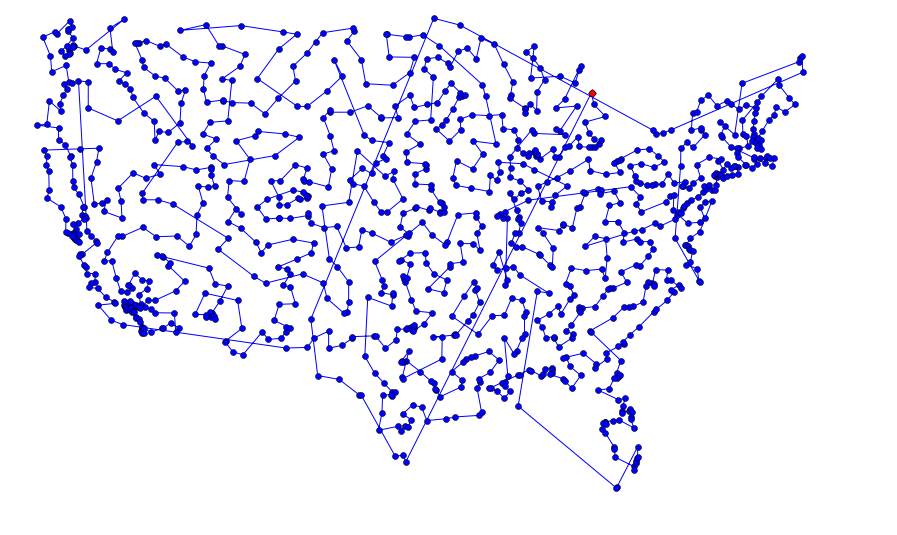There are some obviously bad (long) links in this tour. To understand the problem and how we might fix it, consider this simpler tour:

In :
cities10 = {City(x, 1) for x in range(0, 5)} | {City(x - 1.49, 0) for x in range(5, 10)}

do(nn_tsp, cities10)

nn: 10 cities ⇒ tour length 17 (in 0.000 sec)Starting at the upper left, the tour goes to the right, picking up the 5 cities in a line, jogs downward, and continues to the right, picking up the remaining 5 cities. But then it has a long diagonal link back to the start. Once you've seen this type of configuration a few times it becomes clear: any time a tour has an "X" where two links cross, we can shorten the tour by uncrossing the "X".

You can think of uncrossing the X as deleting two links and adding two new ones. Or you can think of it as reversing a segment: this tour visits the bottom 5 cities in left-to-right order; let's reverse that segment and visit them in right-to-left order:

In :
tour = nn_tsp(cities10)

tour[5:10] = reversed(tour[5:10])

plot_tour(tour)
tour_length(tour)

Out:
15.299342436137655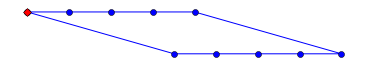That's an improvement!

Below is a diagram to explain why uncrossing the X is always an improvement. Below the crossed lines that we will remove are dotted blue; the lines we will add are dashed red. We can see that two red-and-blue triangles are formed, and by the triangle inequality each red line is shorter than the two parts of the blue lines that make up the rest of the triangle.

In :
plot_tour([tour, tour], 'r--');  plot_tour([tour, tour], 'b:')
plot_tour([tour, tour], 'r--');  plot_tour([tour, tour], 'b:')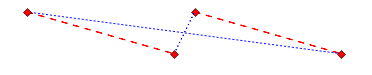It is difficult to get nearest neighbors to avoid mistakes (like crossing an X), because in the middle of the tour, when the algorithm is forced to make a choice, it doesn't know where the rest of the tour will end up. So, rather than tackling the difficult task of avoiding the mistakes, the iterative improvement strategy says to go ahead and make mistakes, finish the tour, and then do the much easier task of fixing the mistakes. Why is it easier to fix the mistakes? Because we can see the whole tour, so we can propose a change and get a definitive answer: either the change makes the whole tour shorter or it doesn't. The strategy is called iterative because we do multiple improvements, if possible.

The changes we propose will all consist of reversing segments; here is how we can check if reversing an arbitrary segment would be an improvement, and if it is, go ahead and do the reversal:

In :
def reverse_segment_if_improvement(tour, i, j):
"If reversing tour[i:j] would make the tour shorter, then do it."
# Given tour [...A,B...C,D...], consider reversing B...C to get [...A,C...B,D...]
A, B, C, D = tour[i-1], tour[i], tour[j-1], tour[j % len(tour)]
# Are old links (AB + CD) longer than new ones (AC + BD)? If so, reverse segment.
if distance(A, B) + distance(C, D) > distance(A, C) + distance(B, D):
tour[i:j] = reversed(tour[i:j])
return True


Now I'll define improve_tour to consider various segments, and reverse the ones that improve the tour. What segments should we consider? I don't know how to be clever about that, but I do know how to use brute force: try every subsegment. (I have an intuition (from experience with simulated annealing) that trying longer subsegments first would be better, so I'll write subsegments that way.) After I've tried all segments, if one of them did improve the tour that might open up new possibilities, so I'll repeat the process until there are no improvements, then return the improved tour:

In :
def improve_tour(tour):
"Try to alter tour for the better by reversing segments."
while True:
improvements = {reverse_segment_if_improvement(tour, i, j)
for (i, j) in subsegments(len(tour))}
if improvements == {None}:

@cache()
def subsegments(N):
"Return (i, j) pairs denoting tour[i:j] subsegments of a tour of length N."
return [(i, i + length)
for length in reversed(range(2, N))
for i in reversed(range(N - length + 1))]


Here are all the subsegments of a 5 city tour:

In :
subsegments(5)

Out:
[(1, 5), (0, 4), (2, 5), (1, 4), (0, 3), (3, 5), (2, 4), (1, 3), (0, 2)]

# Improved Nearest Neighbor Algorithms¶

Here are two ways of improving nearest neighbor algorithms—either improve the single nearest neighbor algorithm, or improve each candidate tour from the repetitive nearest neighbor algorithm:

In :
def improve_nn_tsp(cities):
"Improve the tour produced by nn_tsp."
return improve_tour(nn_tsp(cities))

def rep_improve_nn_tsp(cities, k=5):
"Run nn_tsp from k different starts, improve each tour; keep the best."
return shortest_tour(improve_tour(nn_tsp(cities, start))
for start in sample(cities, k))

In :
do(improve_nn_tsp, cities10)

improve_nn: 10 cities ⇒ tour length 15 (in 0.000 sec)In :
do(improve_nn_tsp, USA)

improve_nn: 1089 cities ⇒ tour length 45489 (in 2.571 sec)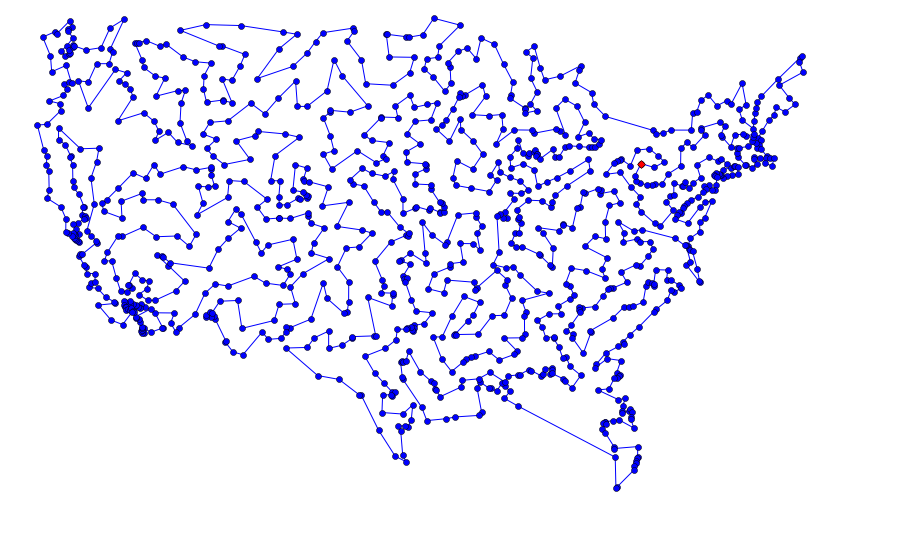Not bad! A single call to improve_tour fixes the cities10 problem, and on the USA map, uncrosses every X and saves over 7000 miles of travel! Let's try repetitions:

In :
do(rep_improve_nn_tsp, USA)

rep_improve_nn: 1089 cities ⇒ tour length 44500 (in 15.181 sec)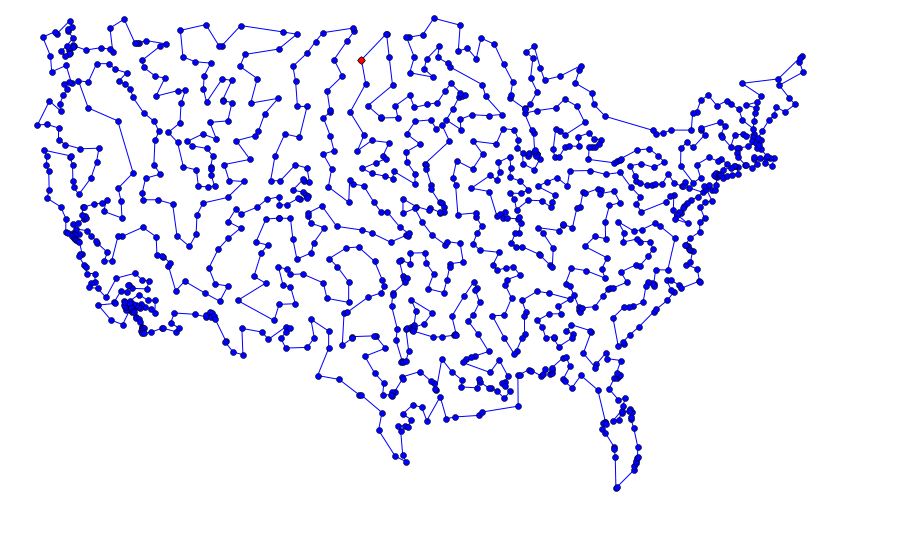Even better! Could we do better still by trying more repetitions? Maybe, but there's a problem: do doesn't accept extra arguments, so I have no place to change rep_improve_nn_tsp's optional k parameter from the default value of 5. I could modify do, but instead I'll define a higher-order function, bind, so that bind(rep_improve_nn_tsp, 5) creates a new function, that, when called with the argument cities, calls rep_improve_nn_tsp(cities, 5). (My bind does the same job as functools.partial, but also sets the function name of the newly created function.)

In :
@cache()
def bind(fn, *extra):
"Bind extra arguments; also assign .__name__"
newfn = lambda *args: fn(*args, *extra)
newfn.__name__ = fn.__name__  + ''.join(', ' + str(x) for x in extra)
return newfn

In :
do(bind(rep_improve_nn_tsp, 10), USA)

rep_improve_nn, 10: 1089 cities ⇒ tour length 44418 (in 32.362 sec)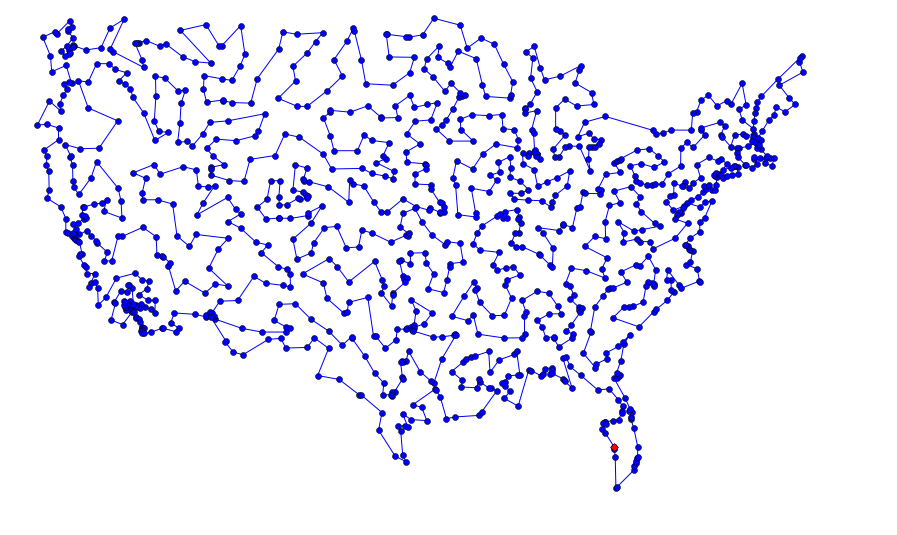We got a slight improvement, but we may be at the point of diminishing returns. Let's try something new.

# Greedy Algorithm: greedy_tsp¶

Let's return to the greedy strategy. We've already covered the Nearest Neighbor Algorithm, which follows the greedy strategy in always choosing the neighbor that is nearest to the last city in the tour. But one problem is that when you get near the end of the tour, you may be left with some very long links. Another way to be greedy is to always grab the shortest possible link, from among all the links between cities. That means we will no longer be building up a single tour, city by city; rather we will be maintaining a set of segments, and joining segments together by greedily choosing the shortest link:

Greedy Algorithm: Maintain a set of segments; intially each city defines its own 1-city segment. Find the shortest possible link that connects two endpoints of two different segments, and join those segments with that link. Repeat until we form a single segment that tours all the cities.

On each step of the algorithm, we want to "find the shortest possible link that connects two endpoints." That seems like an expensive operation to do on each step. So we will add in some data structures to speed up the computation:

1. Pre-compute a list of links, sorted by shortest link first. A link is a pair of cities: (A, B).
2. Maintain a dict that maps endpoints to segments, e.g. {A: [A, B, C], C: [A, B, C], D: [D]} means that A and C are the endpoints of segment [A, B, C] and D is a 1-city segment.
3. Go through the links in shortest-first order. Suppose (B, D) is the next shortest link. We can't use it, because B is already attached to A and C. But if (A, D) is the next shortest, that works: A and D are endpoints of different segments, so we can join the two segments together. Update the endpoints dict to reflect this new segment: {A: [D, A, B, C], D: [D, A, B, C]}.
4. Stop when the newly created segment contains all the cities.

Here is the code:

In :
def greedy_tsp(cities):
"""Go through links, shortest first. Use a link to join segments if possible."""
endpoints = {C: [C] for C in cities} # A dict of {endpoint: segment}
if A in endpoints and B in endpoints and endpoints[A] != endpoints[B]:
new_segment = join_endpoints(endpoints, A, B)
if len(new_segment) == len(cities):
return new_segment

def improve_greedy_tsp(cities): return improve_tour(greedy_tsp(cities))

"Return all links between cities, sorted shortest first."

# TO DO: join_endpoints


Note: The endpoints dict is serving two purposes. First, the keys of the dict are all the cities that are endpoints of some segment, making it possible to ask "A in endpoints" to see if city A is an endpoint. Second, the value of endpoints[A] is the segment that A is an endpoint of, making it possible to ask "endpoints[A] != endpoints[B]" to make sure that the two cities are endpoints of different segments, not of the same segment.

For the join_endpoints function, I first make sure that A is the last element of one segment and B is the first element of the other, by reversing segments if necessary. Then I add the B segment on to the end of the A segment. Finally, I update the endpoints dict by deleting A and B and then adding the two endpoints of the new segment:

In :
def join_endpoints(endpoints, A, B):
"Join segments [...,A] + [B,...] into one segment. Maintain endpoints."
Aseg, Bseg = endpoints[A], endpoints[B]
if Aseg[-1] is not A: Aseg.reverse()
if Bseg  is not B: Bseg.reverse()
Aseg += Bseg
del endpoints[A], endpoints[B]
endpoints[Aseg] = endpoints[Aseg[-1]] = Aseg
return Aseg


Let's try out the greedy_tsp algorithm, with and without improvement, on the USA map:

In :
do(greedy_tsp, USA)

greedy: 1089 cities ⇒ tour length 46982 (in 0.561 sec)